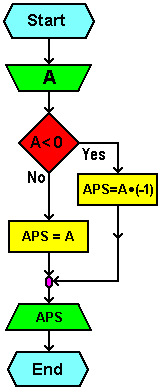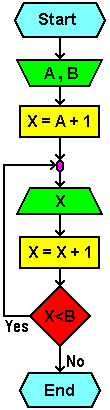## Example VIIWrite an algorithm and flowchart for a program that calculates the absolute value of an integer. Instructions: The absolute value of the distance is an integer from zero. (|-3|=3, |3|=3, |0|=0).

Algorithm:

```start
-----------------------------------------------------
Input A
If A < 0
Then ABSOLUTE = (-1) × A
Else ABSOLUTE = A
Print ABSOLUTE
-----------------------------------------------------
end
```
 Flowchart: Program code:``` CLS CLEAR INPUT "Input A:" A IF A < 0 THEN absl = (-1) * A ELSE absl = A END IF PRINT "Absolutely of A is:" absl END ```## Example VIII

Write algorithm and flow chart for a program that will print every integer in the range between two given numbers A and B. Let us assume that A and B are given by the A+1<B (to print at least one number).

Algorithm:

```start
-----------------------------------------------------
Input A, B
X = A + 1
Print X
X = X + 1
If X < B
Then go back to 'Print X'
Else go to end
-----------------------------------------------------
end
```
 Flowchart: Program code:``` 10 CLS 20 CLEAR 30 INPUT "Input A,B, with A < B" a, b 40 x = a + 1 REM Initial value for X is 0 DO PRINT "The value of X is:" x x = x + 1 REM X increases by 1 LOOP WHILE x < b REM Using 'LOOP UNTIL' at first complied REM the condition goes out of the loop 50 END ```Citing of this page: Radic, Drago. " IT - Informatics Alphabet " Split-Croatia. {Date of access}. . Copyright © by Drago Radic. All rights reserved. | Disclaimer